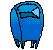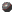## Johann DirichletJohann Peter Gustav Lejeune Dirichlet was the first mathematician to demonstrate that in a mathematical progression with the first term acting as the coprime to the difference, there are an infinite number of prime numbers.

Born on February 13, 1805 in Duren, Germany. In 1826, Dirichlet proved the aforementioned statement which was a conjecture of Karl Gauss. Furthermore, he contributed a great deal to Algebraic number theory and in 1837 proposed the modern definition of a function. The latter was a great accomplishment as it set the parameters for all sorts of modern analytical mathematics. As a consequence, non-Euclidean geometry had less rigid parameters to work from.

Dirichlet is probably most famous for his work on convergence of trigonometric series and the use of arbitrary functions.

The following is Dirichlet's definition of a function:

"If a variable, y, is so related to a variable, x, that whenever a numerical value is assigned to x there is a rule according to which a unique value of y is determined, then y is said to be a function of the independent variable x."

From 1828-1855, Dirichlet taught at the University of Berlin. Thereafter, Dirichlet succeeded the presitgious Gauss as chair of mathematics at Gottingen. Dirichlet died on May 5, 1859 an accomplished mathematician.

Questions or comments, email meFor more information on Dirichlet, click the bulletBack to Famous Mathematicians